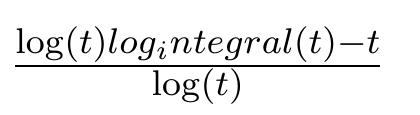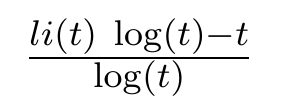# latex problem using result obtained from Fricas integrate

sagemath 8.9.

I do not remember if I mentioned this before or not. I googled and did not find anything. If this is already mentioned and known issue, I am sorry and please feel free to close this.

Fricas returns li in one result, which gets translated log_integral by sagemath. Which is OK. But the latex translation still uses log_integral.

since in Latex, the _ is important , it looks bad when typeset.

So I think the translation of log_integral to latex should be changed to \li or \logintegral. Then one can always make it a math operator using \DeclareMathOperator{\li}{log\_integral} but one can't do this, if the math name itself has _ in it. Latex gives an error.

In non-latex, it is ok to use log_integral.

Here is an example.

sage: var('t')
t
sage: result=integrate(1/log(t)^2,t, algorithm="fricas")
sage: result
(log(t)*log_integral(t) - t)/log(t)
sage: latex(result)
\frac{\log\left(t\right) log_integral\left(t\right) - t}{\log\left(t\right)}


When compiling the above latex, it shows as

\documentclass[11pt]{article}
\usepackage{amsmath}

\begin{document}
$\frac{\log\left(t\right) log_integral\left(t\right) - t}{\log\left(t\right)}$
\end{document}Using Fricas directly

(3) -> result:=integrate(1/log(t)^2,t)

li(t)log(t) - t
(3)  ---------------
log(t)
Type: Union(Expression(Integer),...)
(4) -> latex(result)

(4)
"{{{li \left( {t} \right)} \  {\log \left( {t} \right)}} -t} \over {\log \lef
t( {t} \right)}"
Type: String
(5) ->


Compiling the above gives

\documentclass[11pt]{article}
\usepackage{amsmath}

\begin{document}
${{{li \left( {t} \right)} \ {\log \left( {t} \right)}} -t} \over {\log \left( {t} \right)}$
\end{document}Not perfect either (since 'li should have been \li and made an operator) but it looks better that latex from sagemath,

Is this a known issue? Is there a workaround?

Thank you --Nasser

edit retag close merge delete

A quick workaround:

LatexExpr(latex(result).replace("log_integral","\operatorname{li}"))


Sort by » oldest newest most voted

This is fixed by the definition of log_integral in the library and can't be changed but by changing this definition (i. e. a patch to Sagemath).

Therefore this can be considered as a (minor) defect. Care to report it on Trac and try to fix it yourself ? Look at the Sagemath's developer's guide to have an idea of what this would entail...

more

1